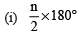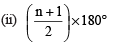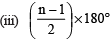Courses

# Multiple Choice Questions- Understanding Quadrilaterals Class 8 Notes | EduRev

## Mathematics (Maths) Class 8

Created by: Indu Gupta

## Class 8 : Multiple Choice Questions- Understanding Quadrilaterals Class 8 Notes | EduRev

The document Multiple Choice Questions- Understanding Quadrilaterals Class 8 Notes | EduRev is a part of the Class 8 Course Mathematics (Maths) Class 8.
All you need of Class 8 at this link: Class 8

Q1. Which of the following quadilaterals has two pairs of adjacent sides equal and diagonals intersecting at right angles?
(i) square
(ii) rhombus
(iii) kite
(iv) rectangle.

Q2.
Which of the following quadrileterals has a pair of opposite sides parallel?

(i) rhombus
(ii) trapezium
(iii) kite
(iv) rectangle.

(i)  rectangle
(ii) square
(iii) rhombus
(iv) kite.

Q4. Which of the quadrilaterals has all angles as right angles, opposite sides equal and diagonals bisect-each other?
(i) rectangle
(ii) rhombus
(iii) square
(iv) none of these.

Q5. Which of the parallelograms has all sides equal and diagonals bisect each other at right angle?
(i) square
(ii) rectangle

(iii) rhombus
(iv) trapezium.

Q6. In an isosceles parallelogram, we have:
(i) pair of parallel sides as equal
(ii) pair of non-parallel sides as equal
(iii) pair of non-parallel sides as perpendicular
(iv) none of these.

Q7. Which of the following is true the adjacent angles of a parallelogram?
(i) they are equal to each other
(ii) they are complementary angles
(iii) they are supplementary angles
(iv) none of these.

Q8. The sides of a pentagon are produced in order. Which of the following is the sum of its exterior angles?
(i) 540°
(ii) 180°

(iii) 720°
(iv) 360°

Q9.
Which of the following is a formula to find the sum of interior angles of a quadrilaters of n-sides?(iv) (n – 2) × 180°

Q10. Diagonals of which of the following quadrilaterals do not bisect it into two congruent tringles?
(i) rhombus
(ii) trapezium
(iii) square
(iv) rectangle.

1. (iii)
2. (ii)
3. (ii)
4. (i)
5. (iii)
6. (ii)
7. (iii)
8. (iv)
9. (iv)
10. (ii)

Offer running on EduRev: Apply code STAYHOME200 to get INR 200 off on our premium plan EduRev Infinity!

,

,

,

,

,

,

,

,

,

,

,

,

,

,

,

,

,

,

,

,

,

;